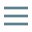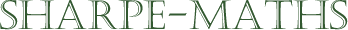# Glossary of terms

## I

•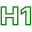### Identities

•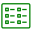Not to be confused with an Identity, identities are statements which are true for all values (the mathematical symbol for for all is $\forall)$.

An identity is identified using a special equals sign with three lines, as below.

•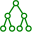$2(x+1) \equiv 2x+2$
•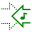•### Identity

•An identity is term from group theory describing an operation which does not change the value of the number being operated upon.

•Under addition, the identity is $0$ because any number $n + 0 = n$.

Under multiplication, the identity is $1$ because any number $n \times 1 = n$.

••### iff

•iff means If and only if. Just a bit stronger than an ordinary if.

•A set of numbers have a product of $0$, iff one or more of the set of numbers is $0$.

••### Imaginary numbers

•Imaginary numbers ($\mathbb{C}$) are numbers based around multiples of $i$, where $i=\sqrt{-1}$.

•$\sqrt{-9} = \sqrt{9} \times \sqrt{-1} = 3i$

In this calculation, $3i$ is an imaginary number.

••### Index (order/power/exponent)

••••### Integers ($\Bbb{Z}$)

•Integers are all of the counting numbers ($\Bbb{N} = \Bbb{Z}^+$) as well as the negative whole numbers ($\Bbb{Z}$-) and zero ($0$). So any whole number on the real number line, positive or negative or zero, is an integer.

•$\{-4, -3, -2, -1, 0, 1, 2, 3, 4\} \in \Bbb{Z}$
••### Irrational numbers

•An irrational number is one which cannot be written as a fraction (integer $\div$ integer).

•$\sqrt{2}$ is irrational.

$\pi$ is irrational

•